# Continuous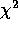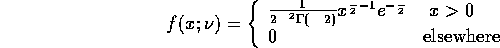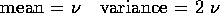(note, the degrees of freedom,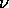is a positive integer)

This distribution is a special case of the gamma distribution (for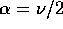and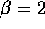). It has application in hypothesis testing.

Beta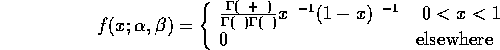(note,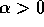and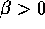)

Cauchy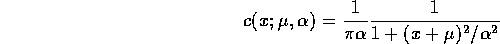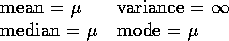Note: the mean of the Cauchy distribution actually does not exist strictly, but since the distribution is symmetric, it is generally taken to be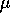.

Erlang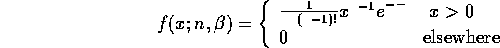(note, n is a positive integer and)

This is a special case of the gamma distribution (where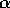is an integer). This distribution has applications in problems concerning the time between independent events, which each have exponential distribution.

Exponential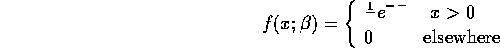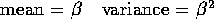(note,)

This distribution has applications in queueing problems, lifetime of events problems and in some physics problems.

F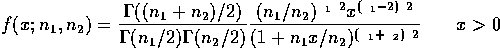(note,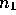and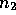are positive integers).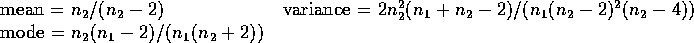Gamma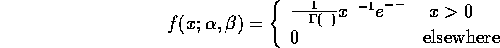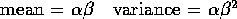(note,and)

Log-Normal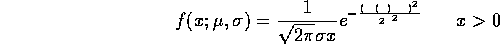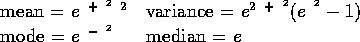Note that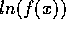is Normal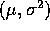, hence the name.

Normal (Gaussian)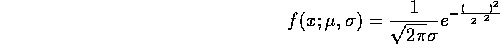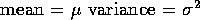Rayleigh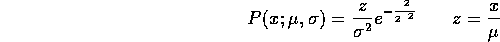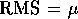Uniform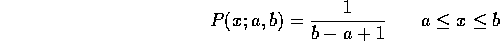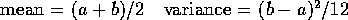Weibull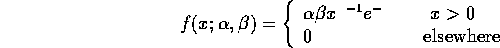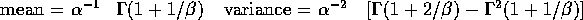(note,and)

Applications in failure, reliability and lifetime problems.

Skip Carter
Wed May 22 15:29:40 PDT 1996